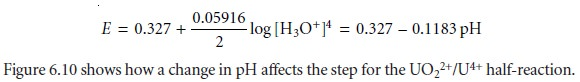Home | | Modern Analytical Chemistry | Ladder Diagram for Oxidation窶迭eduction Equilibria

# Ladder Diagram for Oxidation窶迭eduction Equilibria

Ladder diagrams can also be used to evaluate equilibrium reactions in redox systems.

Ladder diagrams can also be used to evaluate equilibrium reactions in redox sys- tems. Figure 6.9 shows a typical ladder diagram for two half-reactions in which the scale is the electrochemical potential, E. Areas of predominance are defined by the Nernst equation. Using the Fe3+/Fe2+ half-reaction as an example, we writeFor potentials more positive than the standard-state potential, the predominate species is Fe3+, whereas Fe2+ predominates for potentials more negative than Eﾂｰ. When coupled with the step for the Sn4+/Sn2+ half-reaction, we see that Sn2+ can be used to reduce Fe3+. If an excess of Sn2+ is added, the potential of the resulting solu- tion will be near +0.154 V.

Using standard-state potentials to construct a ladder diagram can present problems if solutes are not at their standard-state concentra- tions. Because the concentrations of the reduced and oxidized species are in a logarithmic term, deviations from standard-state concentra- tions can usually be ignored if the steps being compared are separated by at least 0.3 V.1b A trickier problem occurs when a half-reaction窶冱 po- tential is affected by the concentration of another species. For example, the potential for the following half-reactiondepends on the pH of the solution. To define areas of predominance in this case, we begin with the Nernst equationand factor out the concentration of H3O+.From this equation we see that the areas of predominance for UO22+ and U4+ are defined by a step whose potential isStudy Material, Lecturing Notes, Assignment, Reference, Wiki description explanation, brief detail
Modern Analytical Chemistry: Equilibrium Chemistry : Ladder Diagram for Oxidation窶迭eduction Equilibria |